# 5 Ways to Increment the Cell Values in Excel

Microsoft Excel is a powerful tool that is widely used by accountants and finance people to make spreadsheets, reports, sales record, summaries and etc. Regardless of the complexity of task, ‘Serial Number’ is a column which is included in almost all types of worksheets. Sequential serial numbers are usually incremented by 1. None of us like filling this column manually by typing 1, 2, 3 … 1000.  How relaxing is the idea of auto-incrementing the serial number values is. Today, we will share some unique ways of adding serial numbers in your excel workbook.

## 1-    Use Simple Drag:

Let us start with the most simple and common way of filling up the column. In the serial number column:

• Type the initial and second value in the first and second cell, respectively.
• Select both the cells and they will be highlighted.
• Move your cursor to the bottom-right of the cells (a plus sign will appear).
• Drag it down till the end of your column.
• All the values will be filled in a sequence automatically.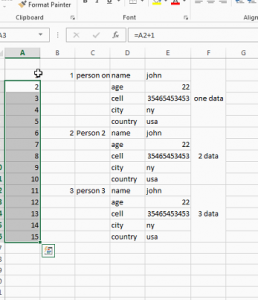## 2-    Auto-increment using User-Defined Formula:

You may also be familiar with another simple technique of incrementing serial numbers. We type a user defined formula that takes the previous cell’s address and add 1 to it.

A2=A1+1

Move the cursor down in the column and press Ctrl + D to copy the formula with appropriate reference cells. You may add any value instead of ‘1’ to generate the next number.

## 3-    Auto – Increment using CountA Function:

We are done with the simple and popular ways of filling up the serial number column. We can also perform the same task using the built-in functions. CountA is a function that counts the number of cells in a range that are not empty. The syntax is very simple. Just type CountA, mention the range of cells and it will calculate the number of items.  In our example, we are counting the number of products being entered by the user. B2 will be the starting cell address for the range of Products. The last value varies with the position of your cursor. It is one less than your current cell address. We have typed the following formula in the serial number cell that corresponds with the first product.

COUNTA(\$B\$2:B3)

‘\$’ sign freezes the cell value. i.e., starting address of the range will now remain the ‘B2’. If you remove the ‘\$’ sign, the entire trick fails as it would not be able to locate the starting point of range correctly.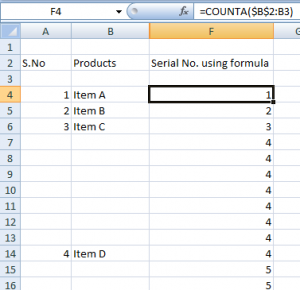You may easily observe the loophole of using CountA. It inserts the serial numbers corresponding to empty cells also. So, we can add a condition that makes sure that serial number is filled only w.r.t some product else it is left blank. We modified the formula as:

=IF(B3=””,””,COUNTA(\$B\$3:B3))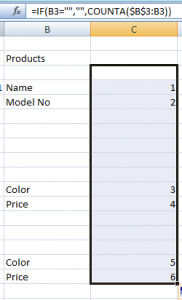## 4-    Use If with the Library functions:

You might have noticed that above mentioned practices fill up the column when you insert the formula for incrementing the values. They do not check whether there is some data associated with the serial number or not. Mostly, we want to assign a serial number as soon as we input any data. We can apply a trick to assign serial numbers using the ‘if’ condition which will insert serial number when data is inputted. This flawless approach will automatically adjust the serial numbers when you delete any item from anywhere in the list.

We will calculate the difference between total number of filled and empty cells in a specified range. Add ‘1’ to this difference to obtain the current serial number. In the serial number column, copy this formula:

=if (B3=””,””, COUNTA (\$A\$2:A2) +1-COUNTBLANK (\$A\$2:A2))

Where,

B3 is the initial cell address for the Products column.

\$A\$2 is the cell address of Serial Number column.

CountA is a function that counts the number of cells in a range that are not empty.

CountBlank is a function that counts the number of empty cells in a specified range of cells.

This formula can be taken as a different flavor of the previous formula.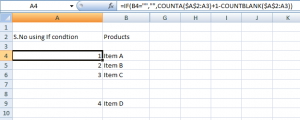## 5-    Increment when New Record Begins:

While working with records we came across data sets where we need to store different attributes that relate to a single object or item. For example, if you are storing the record of any home product, say telephone, it will have name, model number, color and price. In such cases, serial numbers should be incremented when a new record appears. We can use any one of the attributes to specify the beginning of a new record. The selected attribute will be used in the logical test of ‘If Condition’. In our example, we are using incrementing the serial number when ‘Name’ appears in the ‘Products’ column.

=IF(C4=”name”, COUNTA(\$B\$2:B3)+1-COUNTBLANK(\$B\$2:B3),””)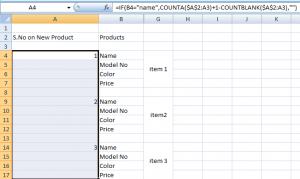So, this was all for today. We hope that you find these tricks handy and helpful. Stay tuned to master your skills at excel!